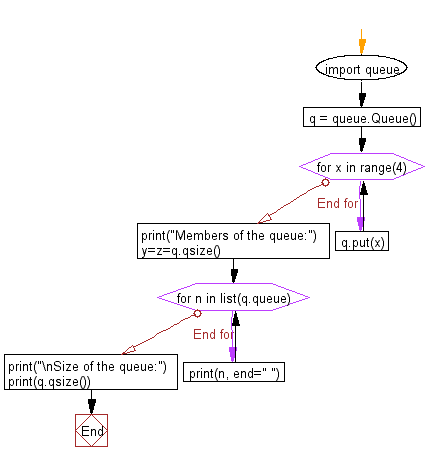﻿ Python heap queue algorithm: Create a queue and display all the members and size of the queue - w3resource

# Python: Create a queue and display all the members and size of the queue

## Python heap queue algorithm: Exercise-26 with Solution

Write a Python program to create a queue and display all the members and the size of the queue.

Sample Solution:

Python Code:

``````import queue
q = queue.Queue()
for x in range(4):
q.put(x)
print("Members of the queue:")
y=z=q.qsize()

for n in list(q.queue):
print(n, end=" ")
print("\nSize of the queue:")
print(q.qsize())
```
```

Sample Output:

```Members of the queue:
0 1 2 3
Size of the queue:
4
```

Flowchart:Python Code Editor:

Have another way to solve this solution? Contribute your code (and comments) through Disqus.

Next: Write a Python program to find whether a queue is empty or not.

What is the difficulty level of this exercise?

Test your Programming skills with w3resource's quiz.

﻿